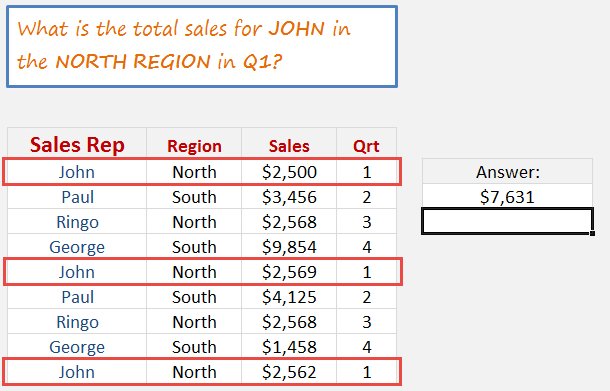What does it do?

It returns the sum of multiple criteria from the corresponding ranges or arrays.

Formula breakdown:

=SUMPRODUCT((array 1 criteria) * (array2 criteria) * array values)

What it means:

=SUMPRODUCT((find my criteria in this array) * (find my criteria in that array) * return the values from the values array)

The SUMPRODUCT function is my favorite Excel function by a stretch!

#### You can create some powerful calculations with the EXCEL SUMPRODUCT function by creating a criteria for a selected array.

For example, you can see how much sales your sales rep did in a particular region and for a particular quarter without having to create a Pivot Table.It takes some practice to get comfortable with Excel SUMPRODUCT multiple criteria function but when you master it, it opens up another Excel world!

Let’s understand how to use this function to sumproduct multiple columns.In our example, we want to get the total sales of John in the North Region in Q1: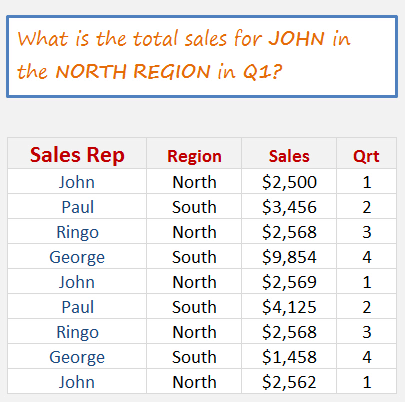STEP 1: We need to enter the SUMPRODUCT Excel function:

## +SUMPRODUCT(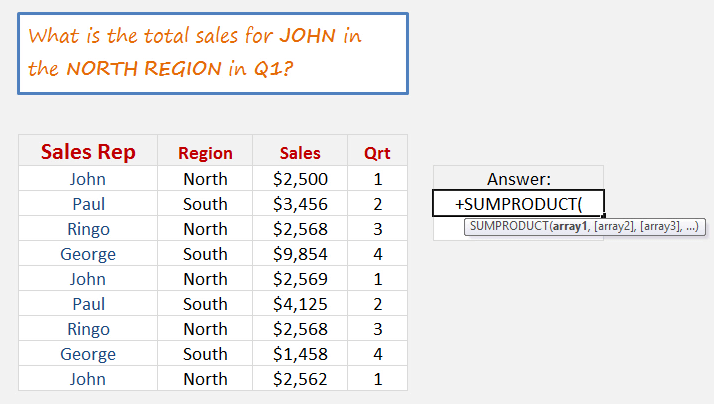STEP 2: Create the criteria for the Sales Rep “John”:

## +SUMPRODUCT((B15:B23=”john”)*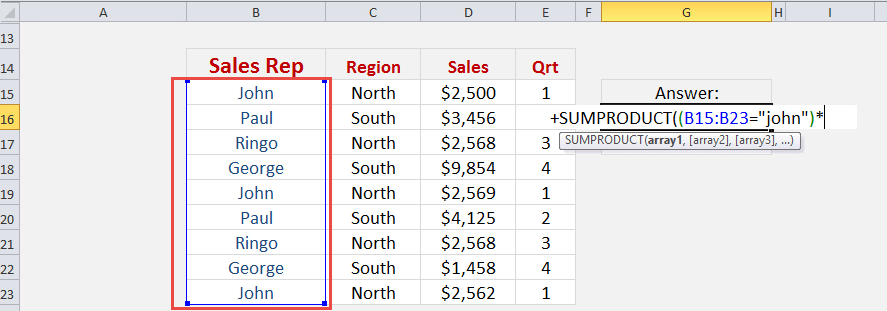Create the criteria for the Region “North”:

## +SUMPRODUCT((B15:B23=”john”)*(C15:C23=”north”)*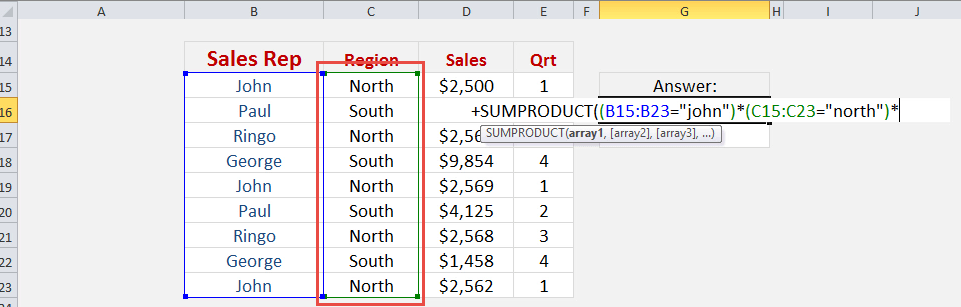Create the criteria for the Quarter “1”:

## +SUMPRODUCT((B15:B23=”john”)*(C15:C23=”north”)*(E15:E23=1)*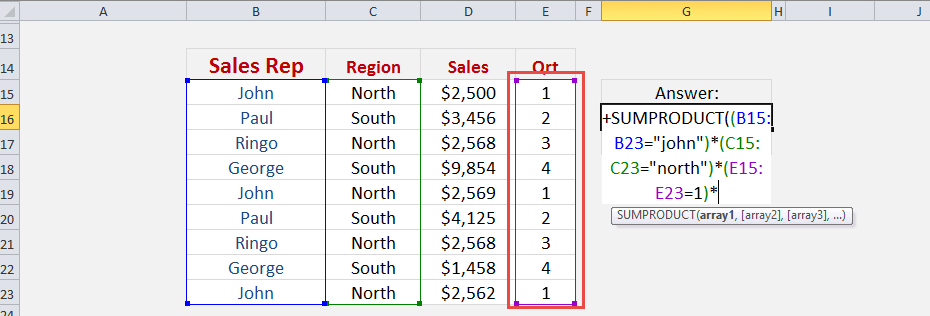Create the sum array to total the values of the Sales column:

## +SUMPRODUCT((B15:B23=”john”)*(C15:C23=”north”)*(E15:E23=1)*D15:D23)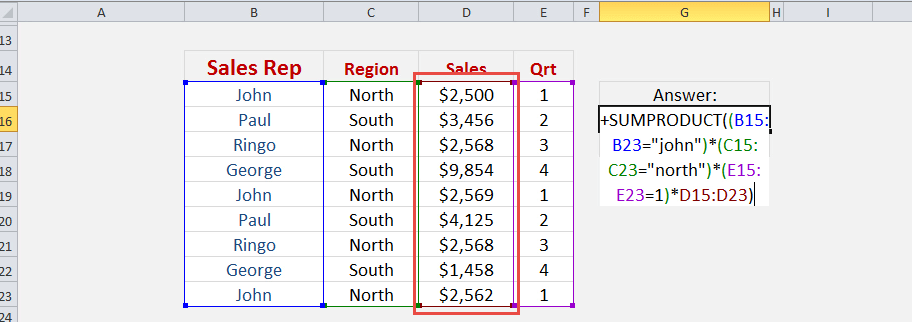Once your formula is complete, you can see that it magically calculated the sum of the matching values! So, this is how you can use sumproduct with multiple criteria.